# xt Problem 9 Suppose that the economy's production function is given by where Y is output,...xt Problem 9 Suppose that the economy's production function is given by where Y is output, K is capital, and N is the number of workers. The steady-state level of capital per worker in terms of the saving rate, s, and the depreciation rate, 8, is ON=□ Property format your oxpression using the too sinthe palette Hover over tools t see keyboard shortcuts. E character) as person t can be Enter your arawer in the answer box and then click Check Answer parts Ciedr All Check Answer MacBook Air 3 4 5 7 K.

Y/N =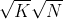Y/N =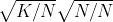Y/N =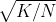.

The following equation shows capital per worker from year t to year (t+1)

Kt+1/N - Kt/N = (s) f(K*/N) - (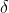) (K*/N)

Under steady state, the capital stock does not change, so Kt+1/N - Kt/N = 0.

(s) f(K*/N) - () (K*/N) = 0

(s) f(K*/N) = () (K*/N)

s *=(K/N)

Squaring both sides,

s2 (K/N) =2 (K/N)2

s2/2 = (K/N).

#### Earn Coin

Coins can be redeemed for fabulous gifts.

Similar Homework Help Questions
• ### Hi These are the correct answers. I would just like help on how to do the...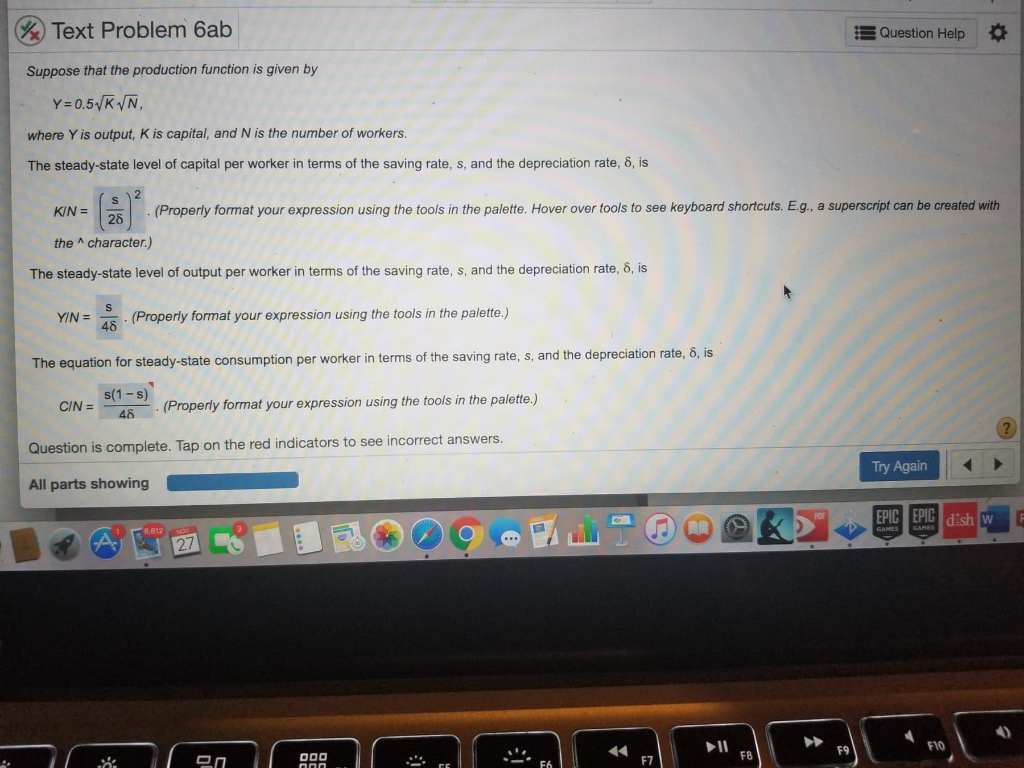Hi These are the correct answers. I would just like help on how to do the math part. I will appreciate your help. Text Problem hab 3 Question Help Suppose that the production function is given by Y=0.5 KN, where Y is output, Kis capital, and N is the number of workers. The steady-state level of capital per worker in terms of the saving rate, s, and the depreciation rate, 8, is KIN= (Properly format your expression using the tools...

• ### Consider the following Cobb Douglas production function, where =0.5 Q=KLP Find the equation for the isoquant...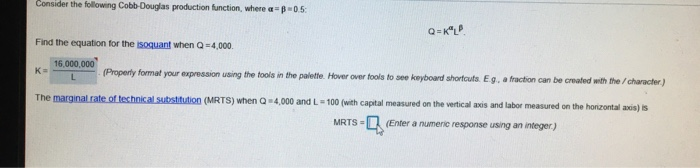Consider the following Cobb Douglas production function, where =0.5 Q=KLP Find the equation for the isoquant when Q=4,000 16.000.000 (Property format your expression using the tools in the palette. Hover over fools to see keyboard shortcuts. Eg, a fraction can be created with the character.) The marginal rate of technical substitution (MRTS) when Q ) 4,000 and L - 100 (with capital measured on the vertical axis and labor measured on the horizontal 2 MRTS - (Enter a numeric response...

• ### 2. that the production function is given by: Suppose where Yis output, K is capital and...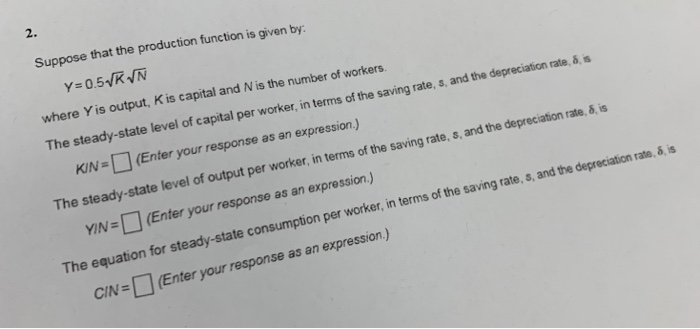2. that the production function is given by: Suppose where Yis output, K is capital and N is the number of workers. The steady-state level of capital per worker, in terms of the saving rate, s., and the depreciation rate, ,s KIN(Enter your response as an expression.) YIN=□ (Enter your response as an expression.) CIN=□ (Enter your response as an expression ) The steady-state level of output per worker, in terms of the saving rate, s, and the depreciation rate,...

• ### Suppose the production function is given by ? = ?^a?'^1-a, where Y is output; K is...

Suppose the production function is given by ? = ?^a?'^1-a, where Y is output; K is capital stock and N is Labor (look Appendix in Chapter 16). a. Is this production function characterized by constant return to scale? How? Show the work. b. Write this production function as a relationship between output per worker and capital per worker. c. If saving (S) equals investment (I), and S = sY, where s is saving rate, what is the corresponding investment per...

• ### David consumes two things: gasoline (9,) and bread (2) David's utility function is U14,4)=104,054205 Let the...David consumes two things: gasoline (9,) and bread (2) David's utility function is U14,4)=104,054205 Let the price of gasoline bep, the price of bread be py, and income be Y. ces Derive David's demand curve for gasoline David's demand for gasoline is (Property format your expression using the fools in the palette Hover over tools to see keyboard shortcuts Eq. a subscript can be created with the character) itions

• ### Consider the following Cobb-Douglas production function, where α Find the equation for the isoquant when Q...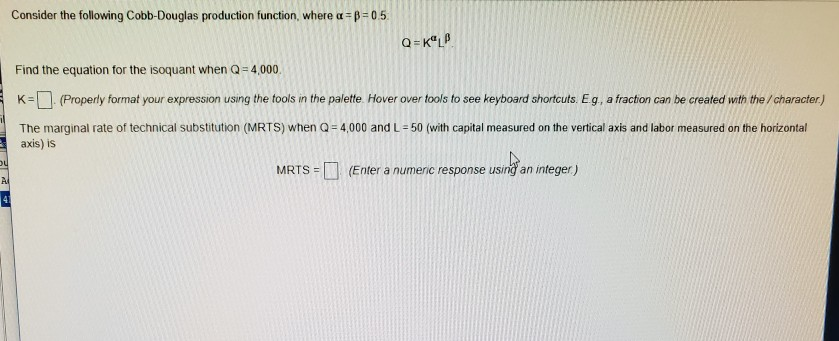Consider the following Cobb-Douglas production function, where α Find the equation for the isoquant when Q 4,000. K-(Properly format your expression using the fools in the paiette. Hover over tools to see keyboard shortcuts Eg, a fraction can be created with the /character) The marginal rate of technical substitution (MRTS) when Q 4,000 and L-50 (with capital measured on the vertical axis and labor measured on the horizontal axis) is MRTS (Enter a numenic response using an integer)

• ### Suppose the production function is given by yt=kt1/2  where y is the output per worker and k...

Suppose the production function is given by yt=kt1/2  where y is the output per worker and k is the capital per worker. Assume that the saving rate (S) is exogenous and the capital depreciates at δ rate. If the sum of both the depreciation rate and saving rate equals 1. Furthermore, assume that S=3δ. The steady state output is

• ### 3. If the production function is given by y=k:13 where yt where yt is the output...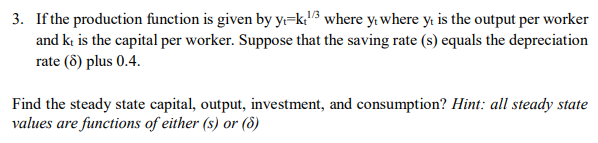3. If the production function is given by y=k:13 where yt where yt is the output per worker and kt is the capital per worker. Suppose that the saving rate (s) equals the depreciation rate (8) plus 0.4. Find the steady state capital, output, investment, and consumption? Hint: all steady state values are functions of either (s) or (8)

• ### Pls solve both of these question pls Hofmework re: 0.5 of 1 pt 12 of 25...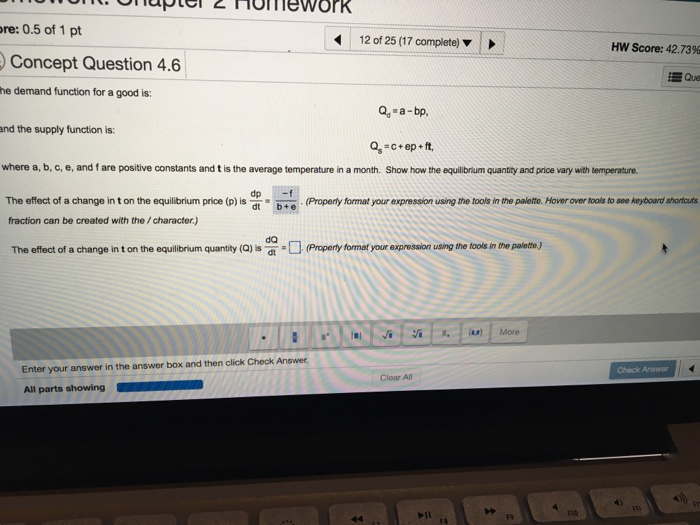Pls solve both of these question pls Hofmework re: 0.5 of 1 pt 12 of 25 (17 complete) Hw Score: 42.73% Concept Question 4.6 Que he demand function for a good is: Q -a-bp and the supply function is: q-c+ep + ft, where a, b, c, e, and f are positive constants and t is the average temperature in a month. Show how the equilibrium quantity and price vary with temperature The effect of a change in t on the...

• ### Consider the Solow growth model. Output at time t is given by the production function Y-AK3...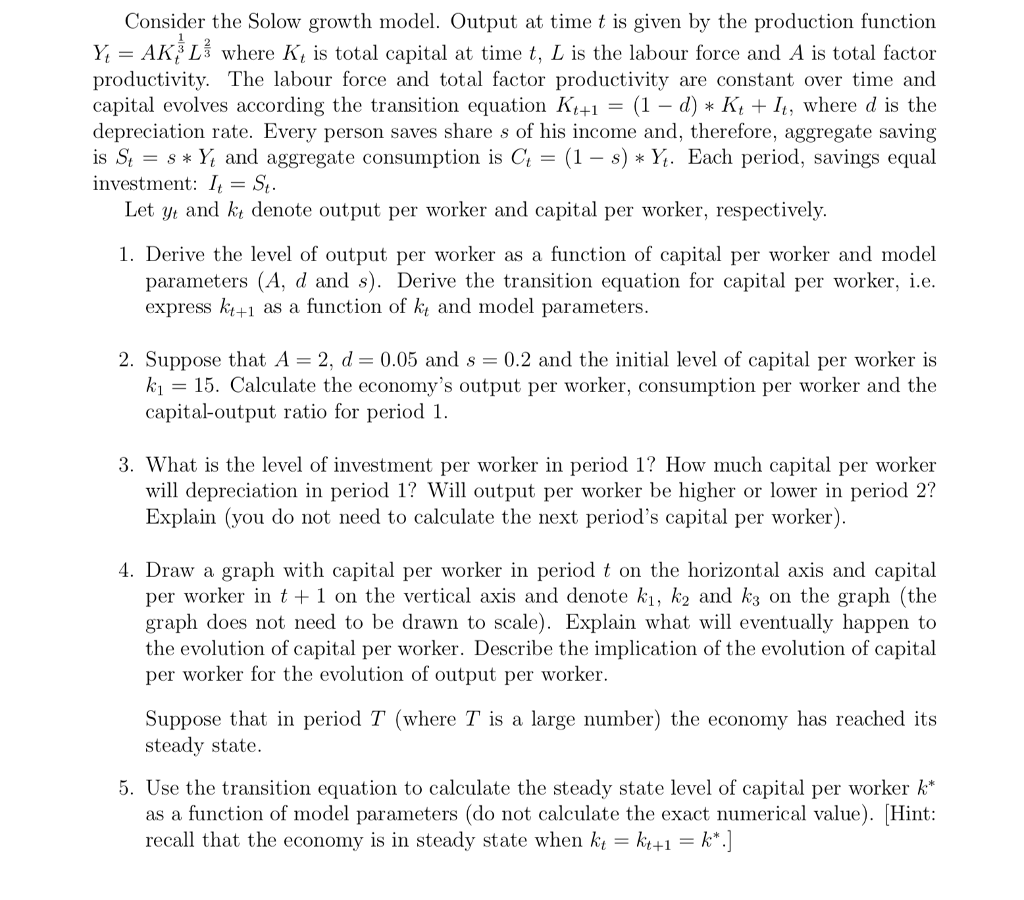Consider the Solow growth model. Output at time t is given by the production function Y-AK3 Lš where K, is total capital at time t, L is the labour force and A is total factor productivity. The labour force and total factor productivity are constant over time and capital evolves according the transition equation KH = (1-d) * Kit It: where d is the depreciation rate. Every person saves share s of his income and, therefore, aggregate saving is St-s...